# Test: Trigonometry- 1

## 10 Questions MCQ Test SSC CGL Tier 2 - Study Material, Online Tests, Previous Year | Test: Trigonometry- 1

Description
Attempt Test: Trigonometry- 1 | 10 questions in 20 minutes | Mock test for SSC preparation | Free important questions MCQ to study SSC CGL Tier 2 - Study Material, Online Tests, Previous Year for SSC Exam | Download free PDF with solutions
QUESTION: 1

### A student is standing with a banner at the top of a 100 m high college building. From a point on the ground, the angle of elevation of the top of the student is 60° and from the same point, the angle of elevation of the top of the tower is 45°. Find the height of the student.

Solution: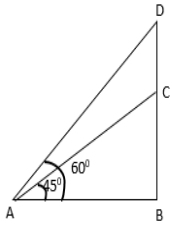Let BC be the height of the tower and DC be the height of the student.
In rt. ΔABC,
AB = BC cot 45° = 100 m

In rt. ΔABD, AB = BD cot 60° = (BC + CD) cot 60° = (10 + CD) * (1 / √3)
∵ AB = 100 m
⇒ (10 + CD) * 1 / √3 = 100
⇒ (10 + CD) = 100√3
⇒ CD = 100√3 - 100 = 100 (1.732 - 1) = 100 x 0.732 = 73.2 m

QUESTION: 2

### If sin (A + B) = √3 / 2 and tan (A – B) = 1. What are the values of A and B?

Solution: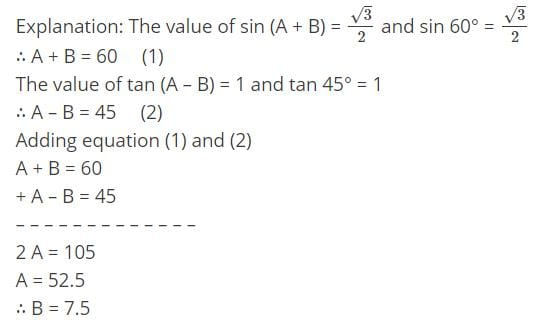QUESTION: 3

### If Cos x – Sin x = √2 Sin x, find the value of Cos x + Sin x:

Solution:

Cos x – Sin x = √2 Sin x
⇒ Cos x = Sin x + √2 Sin x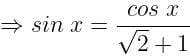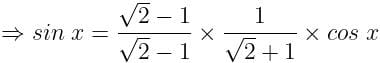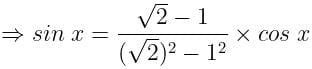⇒ Sin x = (√2 - 1) Cos x
⇒ Sin x = √2 Cos x - Cos x
⇒ Sin x + Cos x = √2 Cos x

QUESTION: 4

If tanØ + sinØ = m, tanØ - sinØ = n, find the value of m2 - n2.

Solution:

Adding the two equations, tanØ = (m + n) / 2
Subtracting the two equations, sinØ = (m - n) / 2

Since, there are no available direct formula for relation between sinØ tanØ.
But we know that: cosec2Ø - cot2Ø = 1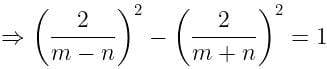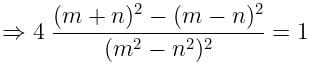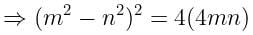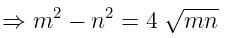QUESTION: 5

If cos A + cos2 A = 1 and a sin12 A + b sin10 A + c sin8 A + d sin6 A - 1 = 0. Find the value of a+b / c+d

Solution:

Cos A = 1 - Cos2A
⇒ Cos A = Sin2A
⇒ Cos2A = Sin4A
⇒ 1 – Sin2A = Sin4A
⇒ 1 = Sin4A + Sin2A
⇒ 13 = (Sin4A + Sin2A)3
⇒ 1 = Sin12A + Sin6A + 3 Sin8A + 3 Sin10A
⇒ Sin12A + Sin6A + 3 Sin8A + 3 Sin10A – 1 = 0

On comparing,
a = 1, b = 3 , c = 3 , d = 1
⇒ (a+b)/(c+d) = 1

QUESTION: 6

3sinx + 4cosx + r is always greater than or equal to 0. What is the smallest value ‘r’ can to take?

Solution: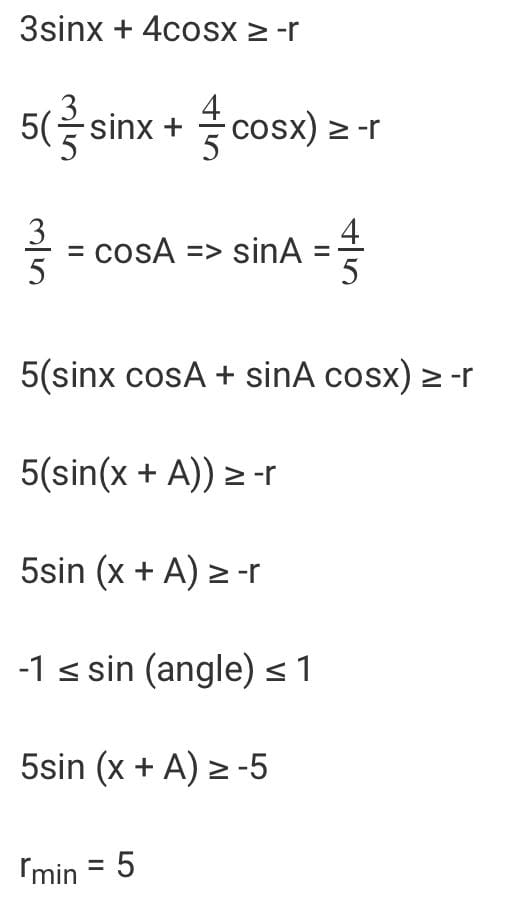Therefore, the answer is Option A.

QUESTION: 7

A right angled triangle has a height ‘p’, base ‘b’ and hypotenuse ‘h’. Which of the following value can h2 not take, given that p and b are positive integers?

Solution:

We know that,
h2 = p2 + b2 Given, p and b are positive integer, so h2 will be sum of two perfect squares.

We see
a) 72 + 52 = 74
b) 62 + 42 = 52
c) 32 + 22 = 13
d) Can’t be expressed as a sum of two perfect squares

Therefore the answer is Option D.

QUESTION: 8

If Cos x – Sin x = √2 Sin x, find the value of Cos x + Sin x:

Solution:

Cos x – Sin x = √2 Sin x

=> Cos x = Sin x + √2 Sin x
=> Cos x = Sin x + √2 Sin x
=> Sin x = Cosx/(√2+1) * Cos x
=> Sin x = (√2−1)/(√2−1) * 1/(√2+1) * Cos x
=> Sin x = (√2−1)/((√2)2−(1)2)* Cos x
=> Sin x = (√2 - 1) Cos x
=> Sin x = √2 Cos x – Cos x
=> Sin x + Cos x = √2 Cos x

Hence, the correct answer is Option A.

QUESTION: 9

You are standing on the corner of a square whose side length is 25 feet. Standing on the opposite corner from you is a tall tree. The angle of elevation from your position to the top of the tree is exactly 60°. How tall is the tree?

Solution:

First find the distance of the diagonal d along the ground from corner to corner. Using Pythagorean theorem with sides 25 and 25, we get:

252 + 252 = d2

2 × 252 = d2

d = 25√ 2 .

Then to obtain the height h of the tree, use the tangent ratio with angle 60°.

tan 60° = x / (25√ 2 )

√ 3  = x / (25√ 2 )

x = 25√ 2  × √ 3  = 25√ 6

QUESTION: 10

Anil looked up at the top of a lighthouse from his boat and found the angle of elevation to be 30 degrees. After sailing in a straight line 50 m towards the lighthouse, he found that the angle of elevation changed to 45 degrees. Find the height of the lighthouse.

Solution: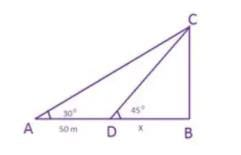If we look at the above image, A is the previous position of the boat. The angle of elevation from this point to the top of the lighthouse is 30 degrees.

After sailing for 50 m, Anil reaches point D from where the angle of elevation is 45 degrees. C is the top of the lighthouse.

Let BD = x

Now, we know tan 30 degrees = 1/ √3 = BC/AB

Tan 45 degrees = 1

=> BC = BD = x

Thus, 1/ √3 = BC/AB = BC / (AD+DB) = x / (50 + x)

Thus x (√3 -1) = 50 or x= 25(√3 +1) mUse Code STAYHOME200 and get INR 200 additional OFF Use Coupon Code